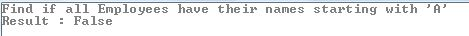# LINQ – Using Quantifiers like Any, Contains and All while Querying Objects

Quantifier operations return a Boolean value if some or all of the elements in a sequence satisfy a condition. In this article, we will see some common LINQ to Objects scenarios where we can use these operators.
There are 3 Quantifiers operations that can be used in LINQ:
All – used to determine whether all the elements in a sequence satisfy a condition.
Any – used to determine whether any elements in a sequence satisfy a condition.
Contains – used to determine whether a sequence contains a specified element
Let us see an example that explains these operators and their usages.
I have created a Department and Employee class. Each Employee belongs to a Department. We will query these objects and retrieve information using LINQ through the quantifiers available to us. Here’s the sample data:
C#
static void Main(string[] args)
{
List<Department> dept = new List<Department>();
dept.Add(new Department() { DeptID = 1, DeptName = “Marketing”, Floor = 1 });
dept.Add(new Department() { DeptID = 2, DeptName = “Sales”, Floor = 2 });
dept.Add(new Department() { DeptID = 3, DeptName = “Administration”, Floor = 3 });
dept.Add(new Department() { DeptID = 4, DeptName = “Accounts”, Floor = 3 });
dept.Add(new Department() { DeptID = 5, DeptName = “HR”, Floor = 3 });
List<Employee> emp = new List<Employee>();
emp.Add(new Employee() { EmpID = 1, DeptID = 1, EmpName = “Jack Nolas”});
emp.Add(new Employee() { EmpID = 2, DeptID = 4, EmpName = “Mark Pine” });
emp.Add(new Employee() { EmpID = 3, DeptID = 3, EmpName = “Sandra Simte” });
emp.Add(new Employee() { EmpID = 4, DeptID = 4, EmpName = “Larry Lo”});
emp.Add(new Employee() { EmpID = 5, DeptID = 3, EmpName = “Sudhir Panj” });
emp.Add(new Employee() { EmpID = 6, DeptID = 2, EmpName = “Kathy K” });
emp.Add(new Employee() { EmpID = 7, DeptID = 1, EmpName = “Kaff Joe” });
emp.Add(new Employee() { EmpID = 8, DeptID = 1, EmpName = “Su Lie” });
}
class Department
{
public int DeptID { get; set; }
public string DeptName { get; set; }
public int Floor { get; set; }
}
class Employee
{
public int EmpID { get; set; }
public int DeptID { get; set; }
public string EmpName { get; set; }
}
Using ‘Any’ Quantifier in LINQ
This sample uses the ‘Any’ operator to list down the Departments that do not have Employees
C#
var noEmp =
from d in dept
where !emp.Any(e => e.DeptID == d.DeptID)
select new { dId = d.DeptID, dNm = d.DeptName };
Console.WriteLine(“Departments having no Employees”);
foreach (var empl in noEmp)
{
Console.WriteLine(“Dept ID – “ + empl.dId + “, Dept Name – “ + empl.dNm);
}
Output:Using ‘Contains’ Quantifier in LINQ
The following example uses the ‘Contains’ quantifier to find the List of Departments having Employee Names starting with ‘S’
C#
// Functionality Similar to IN operator
var hasEmp = dept
.Where(e => emp.Where(contact =>
contact.EmpName.StartsWith(“S”))
.Select(d => d.DeptID)
.Contains(e.DeptID));
Console.WriteLine(“/nList of Departments having Employee Names starting with S”);
foreach (var dpt in hasEmp)
{
Console.WriteLine(“Dept ID – “ + dpt.DeptID + “, Dept Name – “ + dpt.DeptName);
}
Output:
If you want to add another condition to the above query where only Departments in Floor 2 and 3 are to be considered, then here’s how to do so:
C#
List<int> floorNo = new List<int>() {2, 3};
var floo = emp.Where(contact =>
contact.EmpName.StartsWith(“S”))
.Where(du => dept.Where(dp => floorNo.Contains(dp.Floor))
.Select(dp => dp.DeptID)
.Contains(du.DeptID));
Console.WriteLine(“List of Employess with Names starting with S\nand are on Floor 2 or 3”);
foreach (var dpt in floo)
{
Console.WriteLine(“Dept ID – “ + dpt.DeptID + “, Employee Name – “ + dpt.EmpName);
}